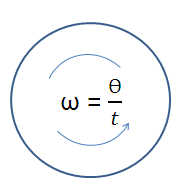# Angular Acceleration Calculator

The calculator finds constant angular acceleration for given values.

 Total torque(in rad/sec) : Mass moment of inertia (in sec):

 Angular Acceleration : (mole)
The rate of change of angular velocity over time is called angular acceleration. In SI units, it is measured in radians per second squared (radians/s2).

## Formula Used:

From Newton's second law

α = T / I

Where,

T = Total torque exerted on the body,

I =Mass moment of inertia of the body

We know that acceleration is the change in velocity of moving object with respect to time, when velocity is changing continuously means object is in acceleration state.

The angular acceleration is zero when angular velocity is constant and if the angular acceleration varies from time to time, then it is refer to average angular acceleration and instantaneous acceleration.